# Textile testing and quality control

2 de Sep de 2020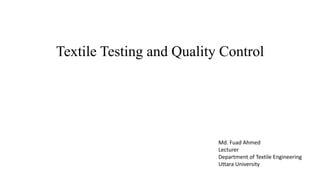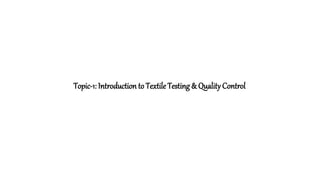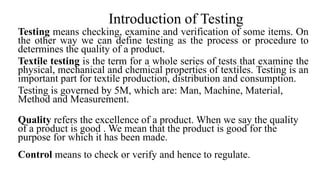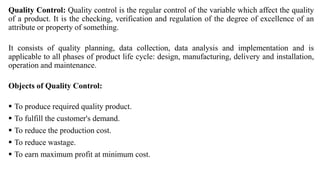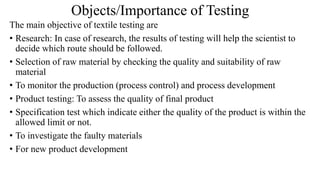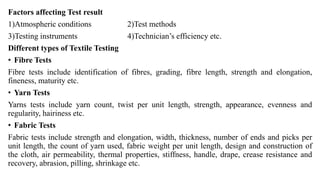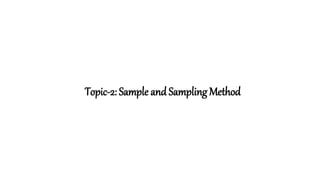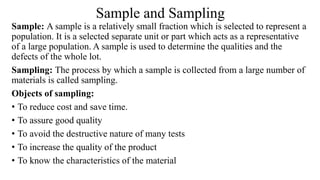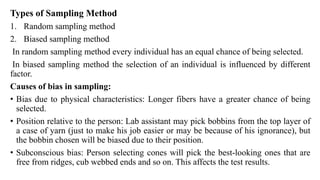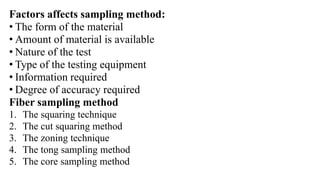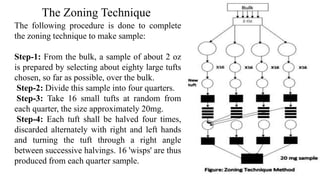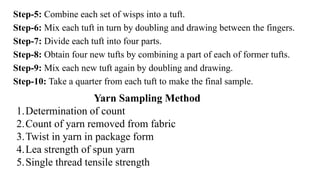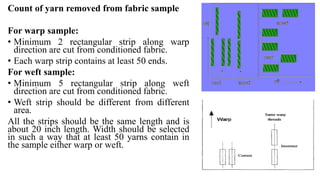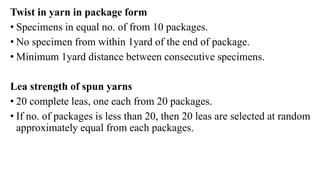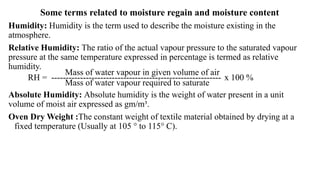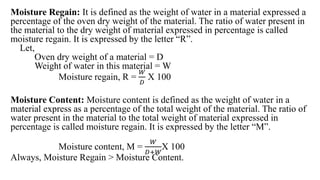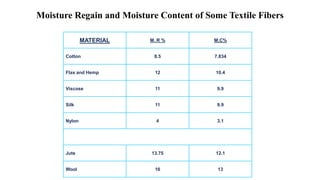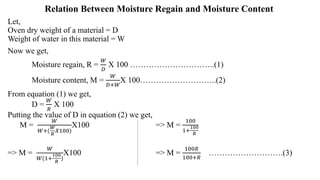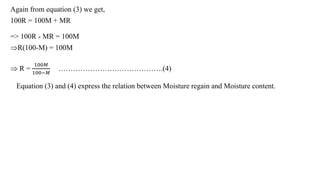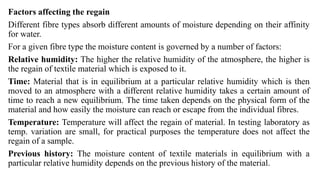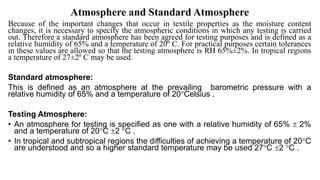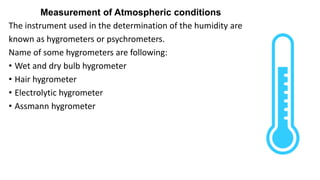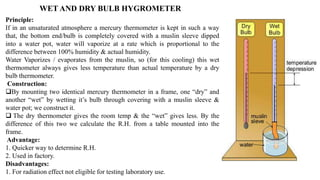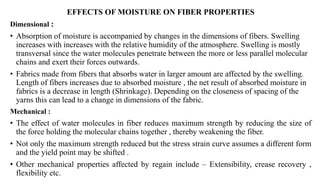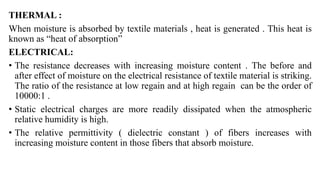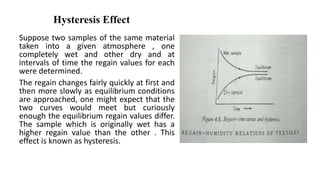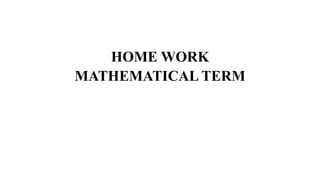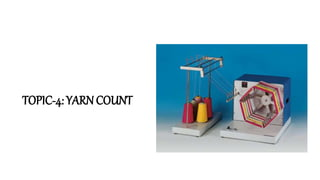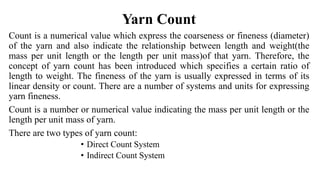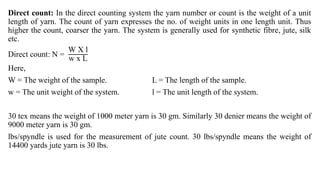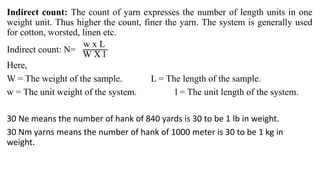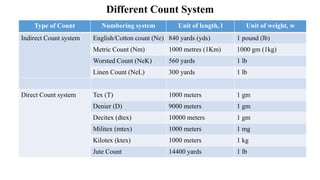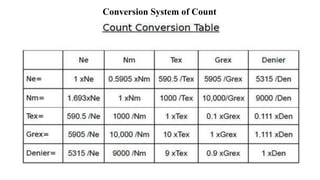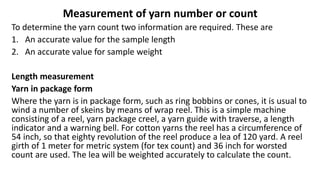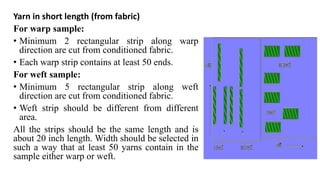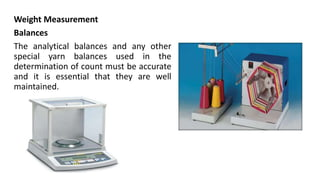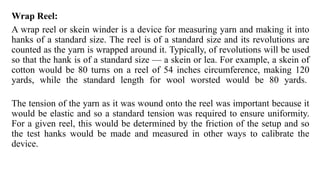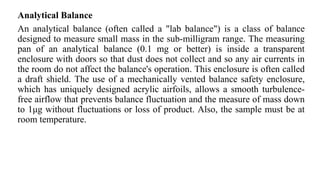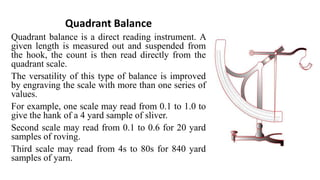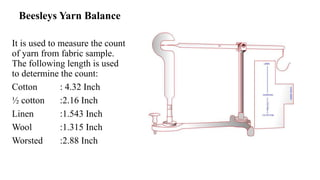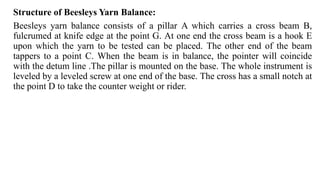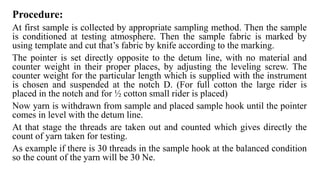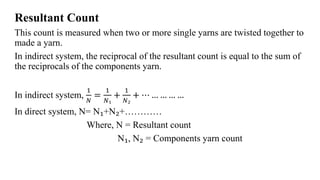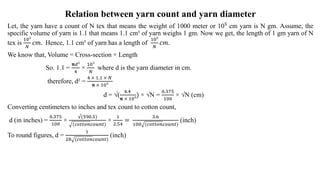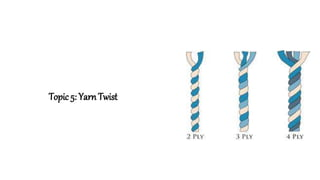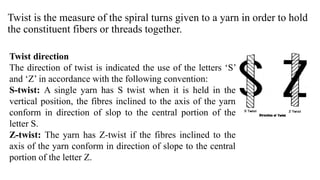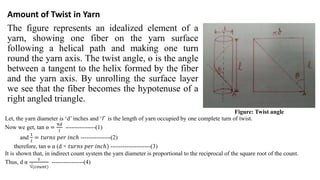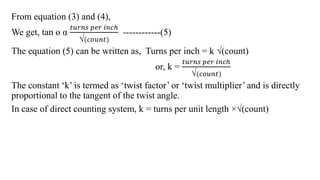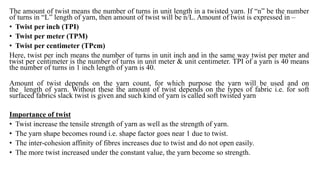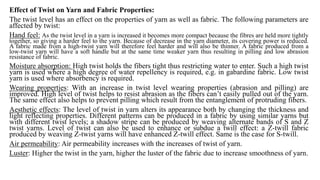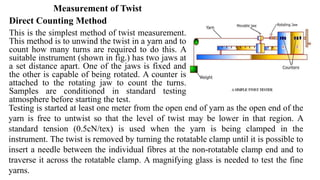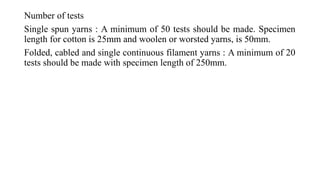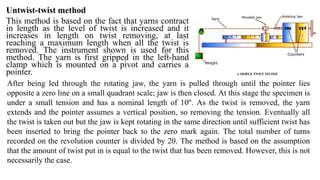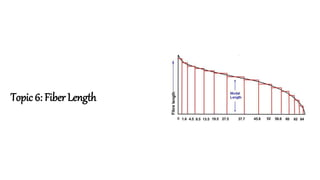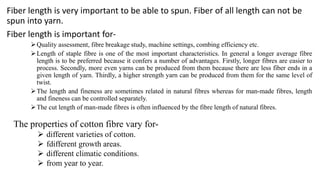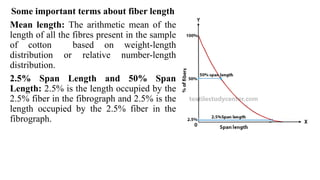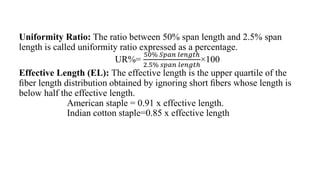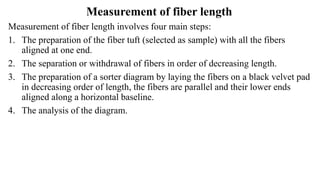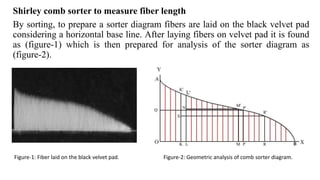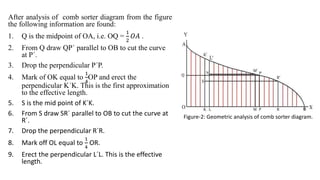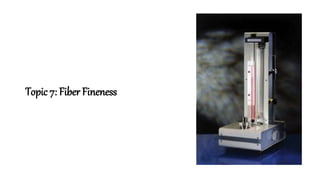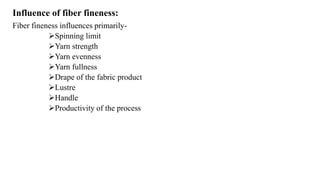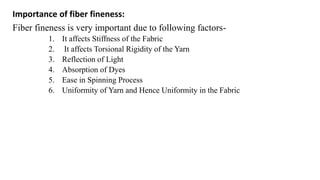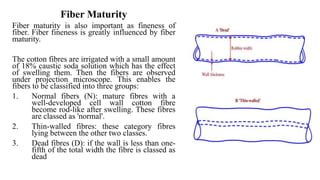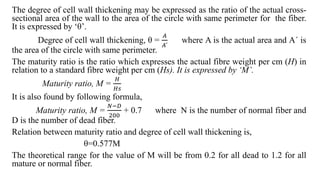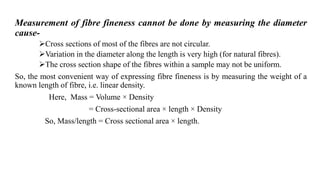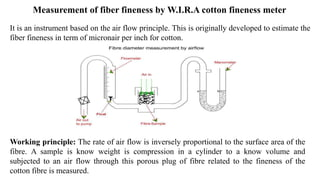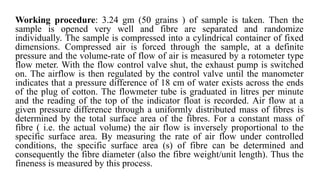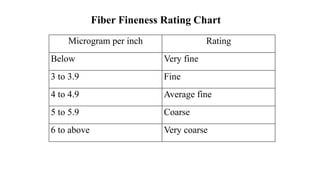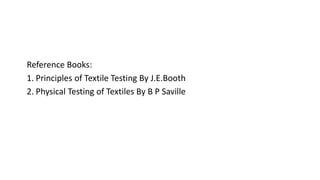1 de 71

### Textile testing and quality control

• 1. Textile Testing and Quality Control Md. Fuad Ahmed Lecturer Department of Textile Engineering Uttara University
• 2. Topic-1: Introduction to Textile Testing & Quality Control
• 3. Introduction of Testing Testing means checking, examine and verification of some items. On the other way we can define testing as the process or procedure to determines the quality of a product. Textile testing is the term for a whole series of tests that examine the physical, mechanical and chemical properties of textiles. Testing is an important part for textile production, distribution and consumption. Testing is governed by 5M, which are: Man, Machine, Material, Method and Measurement. Quality refers the excellence of a product. When we say the quality of a product is good . We mean that the product is good for the purpose for which it has been made. Control means to check or verify and hence to regulate.
• 4. Quality Control: Quality control is the regular control of the variable which affect the quality of a product. It is the checking, verification and regulation of the degree of excellence of an attribute or property of something. It consists of quality planning, data collection, data analysis and implementation and is applicable to all phases of product life cycle: design, manufacturing, delivery and installation, operation and maintenance. Objects of Quality Control:  To produce required quality product.  To fulfill the customer's demand.  To reduce the production cost.  To reduce wastage.  To earn maximum profit at minimum cost.
• 5. Objects/Importance of Testing The main objective of textile testing are • Research: In case of research, the results of testing will help the scientist to decide which route should be followed. • Selection of raw material by checking the quality and suitability of raw material • To monitor the production (process control) and process development • Product testing: To assess the quality of final product • Specification test which indicate either the quality of the product is within the allowed limit or not. • To investigate the faulty materials • For new product development
• 6. Factors affecting Test result 1)Atmospheric conditions 2)Test methods 3)Testing instruments 4)Technician’s efficiency etc. Different types of Textile Testing • Fibre Tests Fibre tests include identification of fibres, grading, fibre length, strength and elongation, fineness, maturity etc. • Yarn Tests Yarns tests include yarn count, twist per unit length, strength, appearance, evenness and regularity, hairiness etc. • Fabric Tests Fabric tests include strength and elongation, width, thickness, number of ends and picks per unit length, the count of yarn used, fabric weight per unit length, design and construction of the cloth, air permeability, thermal properties, stiffness, handle, drape, crease resistance and recovery, abrasion, pilling, shrinkage etc.
• 7. Topic-2: Sample and Sampling Method
• 8. Sample and Sampling Sample: A sample is a relatively small fraction which is selected to represent a population. It is a selected separate unit or part which acts as a representative of a large population. A sample is used to determine the qualities and the defects of the whole lot. Sampling: The process by which a sample is collected from a large number of materials is called sampling. Objects of sampling: • To reduce cost and save time. • To assure good quality • To avoid the destructive nature of many tests • To increase the quality of the product • To know the characteristics of the material
• 9. Types of Sampling Method 1. Random sampling method 2. Biased sampling method In random sampling method every individual has an equal chance of being selected. In biased sampling method the selection of an individual is influenced by different factor. Causes of bias in sampling: • Bias due to physical characteristics: Longer fibers have a greater chance of being selected. • Position relative to the person: Lab assistant may pick bobbins from the top layer of a case of yarn (just to make his job easier or may be because of his ignorance), but the bobbin chosen will be biased due to their position. • Subconscious bias: Person selecting cones will pick the best-looking ones that are free from ridges, cub webbed ends and so on. This affects the test results.
• 10. Factors affects sampling method: • The form of the material • Amount of material is available • Nature of the test • Type of the testing equipment • Information required • Degree of accuracy required Fiber sampling method 1. The squaring technique 2. The cut squaring method 3. The zoning technique 4. The tong sampling method 5. The core sampling method
• 11. The Zoning Technique The following procedure is done to complete the zoning technique to make sample: Step-1: From the bulk, a sample of about 2 oz is prepared by selecting about eighty large tufts chosen, so far as possible, over the bulk. Step-2: Divide this sample into four quarters. Step-3: Take 16 small tufts at random from each quarter, the size approximately 20mg. Step-4: Each tuft shall be halved four times, discarded alternately with right and left hands and turning the tuft through a right angle between successive halvings. 16 'wisps' are thus produced from each quarter sample.
• 12. Step-5: Combine each set of wisps into a tuft. Step-6: Mix each tuft in turn by doubling and drawing between the fingers. Step-7: Divide each tuft into four parts. Step-8: Obtain four new tufts by combining a part of each of former tufts. Step-9: Mix each new tuft again by doubling and drawing. Step-10: Take a quarter from each tuft to make the final sample. Yarn Sampling Method 1.Determination of count 2.Count of yarn removed from fabric 3.Twist in yarn in package form 4.Lea strength of spun yarn 5.Single thread tensile strength
• 13. Count of yarn removed from fabric sample For warp sample: • Minimum 2 rectangular strip along warp direction are cut from conditioned fabric. • Each warp strip contains at least 50 ends. For weft sample: • Minimum 5 rectangular strip along weft direction are cut from conditioned fabric. • Weft strip should be different from different area. All the strips should be the same length and is about 20 inch length. Width should be selected in such a way that at least 50 yarns contain in the sample either warp or weft.
• 14. Twist in yarn in package form • Specimens in equal no. of from 10 packages. • No specimen from within 1yard of the end of package. • Minimum 1yard distance between consecutive specimens. Lea strength of spun yarns • 20 complete leas, one each from 20 packages. • If no. of packages is less than 20, then 20 leas are selected at random approximately equal from each packages.
• 15. Topic-3: Moisture Regain & Moisture Content
• 16. Some terms related to moisture regain and moisture content Humidity: Humidity is the term used to describe the moisture existing in the atmosphere. Relative Humidity: The ratio of the actual vapour pressure to the saturated vapour pressure at the same temperature expressed in percentage is termed as relative humidity. Mass of water vapour in given volume of air RH = ----------------------------------------------------------- x 100 % Mass of water vapour required to saturate Absolute Humidity: Absolute humidity is the weight of water present in a unit volume of moist air expressed as gm/m³. Oven Dry Weight :The constant weight of textile material obtained by drying at a fixed temperature (Usually at 105  to 115 C).
• 17. Moisture Regain: It is defined as the weight of water in a material expressed a percentage of the oven dry weight of the material. The ratio of water present in the material to the dry weight of material expressed in percentage is called moisture regain. It is expressed by the letter “R”. Let, Oven dry weight of a material = D Weight of water in this material = W Moisture regain, R = 𝑊 𝐷 X 100 Moisture Content: Moisture content is defined as the weight of water in a material express as a percentage of the total weight of the material. The ratio of water present in the material to the total weight of material expressed in percentage is called moisture regain. It is expressed by the letter “M”. Moisture content, M = 𝑊 𝐷+𝑊 X 100 Always, Moisture Regain > Moisture Content.
• 18. Moisture Regain and Moisture Content of Some Textile Fibers MATERIAL M. R % M.C% Cotton 8.5 7.834 Flax and Hemp 12 10.4 Viscose 11 9.9 Silk 11 9.9 Nylon 4 3.1 Jute 13.75 12.1 Wool 16 13
• 19. Relation Between Moisture Regain and Moisture Content Let, Oven dry weight of a material = D Weight of water in this material = W Now we get, Moisture regain, R = 𝑊 𝐷 X 100 …………………………..(1) Moisture content, M = 𝑊 𝐷+𝑊 X 100………………………..(2) From equation (1) we get, D = 𝑊 𝑅 X 100 Putting the value of D in equation (2) we get, M = 𝑊 𝑊+( 𝑊 𝑅 𝑋100) X100 => M = 100 1+ 100 𝑅 => M = 𝑊 𝑊(1+ 100 𝑅 ) X100 => M = 100𝑅 100+𝑅 ……………………….(3)
• 20. Again from equation (3) we get, 100R = 100M + MR => 100R - MR = 100M R(100-M) = 100M  R = 100𝑀 100−𝑀 …………………………………….(4) Equation (3) and (4) express the relation between Moisture regain and Moisture content.
• 21. Factors affecting the regain Different fibre types absorb different amounts of moisture depending on their affinity for water. For a given fibre type the moisture content is governed by a number of factors: Relative humidity: The higher the relative humidity of the atmosphere, the higher is the regain of textile material which is exposed to it. Time: Material that is in equilibrium at a particular relative humidity which is then moved to an atmosphere with a different relative humidity takes a certain amount of time to reach a new equilibrium. The time taken depends on the physical form of the material and how easily the moisture can reach or escape from the individual fibres. Temperature: Temperature will affect the regain of material. In testing laboratory as temp. variation are small, for practical purposes the temperature does not affect the regain of a sample. Previous history: The moisture content of textile materials in equilibrium with a particular relative humidity depends on the previous history of the material.
• 22. Atmosphere and Standard Atmosphere Because of the important changes that occur in textile properties as the moisture content changes, it is necessary to specify the atmospheric conditions in which any testing is carried out. Therefore a standard atmosphere has been agreed for testing purposes and is defined as a relative humidity of 65% and a temperature of 20º C. For practical purposes certain tolerances in these values are allowed so that the testing atmosphere is RH 65%±2%. In tropical regions a temperature of 27±2º C may be used. Standard atmosphere: This is defined as an atmosphere at the prevailing barometric pressure with a relative humidity of 65% and a temperature of 20Celsius . Testing Atmosphere: • An atmosphere for testing is specified as one with a relative humidity of 65%  2% and a temperature of 20C 2 C . • In tropical and subtropical regions the difficulties of achieving a temperature of 20C are understood and so a higher standard temperature may be used 27C 2 C .
• 23. Measurement of Atmospheric conditions The instrument used in the determination of the humidity are known as hygrometers or psychrometers. Name of some hygrometers are following: • Wet and dry bulb hygrometer • Hair hygrometer • Electrolytic hygrometer • Assmann hygrometer
• 24. WET AND DRY BULB HYGROMETER Principle: If in an unsaturated atmosphere a mercury thermometer is kept in such a way that, the bottom end/bulb is completely covered with a muslin sleeve dipped into a water pot, water will vaporize at a rate which is proportional to the difference between 100% humidity & actual humidity. Water Vaporizes / evaporates from the muslin, so (for this cooling) this wet thermometer always gives less temperature than actual temperature by a dry bulb thermometer. Construction: By mounting two identical mercury thermometer in a frame, one “dry” and another “wet” by wetting it’s bulb through covering with a muslin sleeve & water pot; we construct it.  The dry thermometer gives the room temp & the “wet” gives less. By the difference of this two we calculate the R.H. from a table mounted into the frame. Advantage: 1. Quicker way to determine R.H. 2. Used in factory. Disadvantages: 1. For radiation effect not eligible for testing laboratory use.
• 25. EFFECTS OF MOISTURE ON FIBER PROPERTIES Dimensional : • Absorption of moisture is accompanied by changes in the dimensions of fibers. Swelling increases with increases with the relative humidity of the atmosphere. Swelling is mostly transversal since the water molecules penetrate between the more or less parallel molecular chains and exert their forces outwards. • Fabrics made from fibers that absorbs water in larger amount are affected by the swelling. Length of fibers increases due to absorbed moisture , the net result of absorbed moisture in fabrics is a decrease in length (Shrinkage). Depending on the closeness of spacing of the yarns this can lead to a change in dimensions of the fabric. Mechanical : • The effect of water molecules in fiber reduces maximum strength by reducing the size of the force holding the molecular chains together , thereby weakening the fiber. • Not only the maximum strength reduced but the stress strain curve assumes a different form and the yield point may be shifted . • Other mechanical properties affected by regain include – Extensibility, crease recovery , flexibility etc.
• 26. THERMAL : When moisture is absorbed by textile materials , heat is generated . This heat is known as “heat of absorption” ELECTRICAL: • The resistance decreases with increasing moisture content . The before and after effect of moisture on the electrical resistance of textile material is striking. The ratio of the resistance at low regain and at high regain can be the order of 10000:1 . • Static electrical charges are more readily dissipated when the atmospheric relative humidity is high. • The relative permittivity ( dielectric constant ) of fibers increases with increasing moisture content in those fibers that absorb moisture.
• 27. Hysteresis Effect Suppose two samples of the same material taken into a given atmosphere , one completely wet and other dry and at intervals of time the regain values for each were determined. The regain changes fairly quickly at first and then more slowly as equilibrium conditions are approached, one might expect that the two curves would meet but curiously enough the equilibrium regain values differ. The sample which is originally wet has a higher regain value than the other . This effect is known as hysteresis.
• 30. Yarn Count Count is a numerical value which express the coarseness or fineness (diameter) of the yarn and also indicate the relationship between length and weight(the mass per unit length or the length per unit mass)of that yarn. Therefore, the concept of yarn count has been introduced which specifies a certain ratio of length to weight. The fineness of the yarn is usually expressed in terms of its linear density or count. There are a number of systems and units for expressing yarn fineness. Count is a number or numerical value indicating the mass per unit length or the length per unit mass of yarn. There are two types of yarn count: • Direct Count System • Indirect Count System
• 31. Direct count: In the direct counting system the yarn number or count is the weight of a unit length of yarn. The count of yarn expresses the no. of weight units in one length unit. Thus higher the count, coarser the yarn. The system is generally used for synthetic fibre, jute, silk etc. Direct count: N = W X l w x L Here, W = The weight of the sample. L = The length of the sample. w = The unit weight of the system. l = The unit length of the system. 30 tex means the weight of 1000 meter yarn is 30 gm. Similarly 30 denier means the weight of 9000 meter yarn is 30 gm. lbs/spyndle is used for the measurement of jute count. 30 lbs/spyndle means the weight of 14400 yards jute yarn is 30 lbs.
• 32. Indirect count: The count of yarn expresses the number of length units in one weight unit. Thus higher the count, finer the yarn. The system is generally used for cotton, worsted, linen etc. Indirect count: N= w x L W X l Here, W = The weight of the sample. L = The length of the sample. w = The unit weight of the system. l = The unit length of the system. 30 Ne means the number of hank of 840 yards is 30 to be 1 lb in weight. 30 Nm yarns means the number of hank of 1000 meter is 30 to be 1 kg in weight.
• 33. Different Count System Type of Count Numbering system Unit of length, l Unit of weight, w Indirect Count system English/Cotton count (Ne) 840 yards (yds) 1 pound (lb) Metric Count (Nm) 1000 metres (1Km) 1000 gm (1kg) Worsted Count (NeK) 560 yards 1 lb Linen Count (NeL) 300 yards 1 lb Direct Count system Tex (T) 1000 meters 1 gm Denier (D) 9000 meters 1 gm Decitex (dtex) 10000 meters 1 gm Militex (mtex) 1000 meters 1 mg Kilotex (ktex) 1000 meters 1 kg Jute Count 14400 yards 1 lb
• 35. Measurement of yarn number or count To determine the yarn count two information are required. These are 1. An accurate value for the sample length 2. An accurate value for sample weight Length measurement Yarn in package form Where the yarn is in package form, such as ring bobbins or cones, it is usual to wind a number of skeins by means of wrap reel. This is a simple machine consisting of a reel, yarn package creel, a yarn guide with traverse, a length indicator and a warning bell. For cotton yarns the reel has a circumference of 54 inch, so that eighty revolution of the reel produce a lea of 120 yard. A reel girth of 1 meter for metric system (for tex count) and 36 inch for worsted count are used. The lea will be weighted accurately to calculate the count.
• 36. Yarn in short length (from fabric) For warp sample: • Minimum 2 rectangular strip along warp direction are cut from conditioned fabric. • Each warp strip contains at least 50 ends. For weft sample: • Minimum 5 rectangular strip along weft direction are cut from conditioned fabric. • Weft strip should be different from different area. All the strips should be the same length and is about 20 inch length. Width should be selected in such a way that at least 50 yarns contain in the sample either warp or weft.
• 37. Weight Measurement Balances The analytical balances and any other special yarn balances used in the determination of count must be accurate and it is essential that they are well maintained.
• 38. Wrap Reel: A wrap reel or skein winder is a device for measuring yarn and making it into hanks of a standard size. The reel is of a standard size and its revolutions are counted as the yarn is wrapped around it. Typically, of revolutions will be used so that the hank is of a standard size — a skein or lea. For example, a skein of cotton would be 80 turns on a reel of 54 inches circumference, making 120 yards, while the standard length for wool worsted would be 80 yards. The tension of the yarn as it was wound onto the reel was important because it would be elastic and so a standard tension was required to ensure uniformity. For a given reel, this would be determined by the friction of the setup and so the test hanks would be made and measured in other ways to calibrate the device.
• 39. Analytical Balance An analytical balance (often called a "lab balance") is a class of balance designed to measure small mass in the sub-milligram range. The measuring pan of an analytical balance (0.1 mg or better) is inside a transparent enclosure with doors so that dust does not collect and so any air currents in the room do not affect the balance's operation. This enclosure is often called a draft shield. The use of a mechanically vented balance safety enclosure, which has uniquely designed acrylic airfoils, allows a smooth turbulence- free airflow that prevents balance fluctuation and the measure of mass down to 1μg without fluctuations or loss of product. Also, the sample must be at room temperature.
• 40. Quadrant Balance Quadrant balance is a direct reading instrument. A given length is measured out and suspended from the hook, the count is then read directly from the quadrant scale. The versatility of this type of balance is improved by engraving the scale with more than one series of values. For example, one scale may read from 0.1 to 1.0 to give the hank of a 4 yard sample of sliver. Second scale may read from 0.1 to 0.6 for 20 yard samples of roving. Third scale may read from 4s to 80s for 840 yard samples of yarn.
• 41. Beesleys Yarn Balance It is used to measure the count of yarn from fabric sample. The following length is used to determine the count: Cotton : 4.32 Inch ½ cotton :2.16 Inch Linen :1.543 Inch Wool :1.315 Inch Worsted :2.88 Inch
• 42. Structure of Beesleys Yarn Balance: Beesleys yarn balance consists of a pillar A which carries a cross beam B, fulcrumed at knife edge at the point G. At one end the cross beam is a hook E upon which the yarn to be tested can be placed. The other end of the beam tappers to a point C. When the beam is in balance, the pointer will coincide with the detum line .The pillar is mounted on the base. The whole instrument is leveled by a leveled screw at one end of the base. The cross has a small notch at the point D to take the counter weight or rider.
• 43. Procedure: At first sample is collected by appropriate sampling method. Then the sample is conditioned at testing atmosphere. Then the sample fabric is marked by using template and cut that’s fabric by knife according to the marking. The pointer is set directly opposite to the detum line, with no material and counter weight in their proper places, by adjusting the leveling screw. The counter weight for the particular length which is supplied with the instrument is chosen and suspended at the notch D. (For full cotton the large rider is placed in the notch and for ½ cotton small rider is placed) Now yarn is withdrawn from sample and placed sample hook until the pointer comes in level with the detum line. At that stage the threads are taken out and counted which gives directly the count of yarn taken for testing. As example if there is 30 threads in the sample hook at the balanced condition so the count of the yarn will be 30 Ne.
• 44. Resultant Count This count is measured when two or more single yarns are twisted together to made a yarn. In indirect system, the reciprocal of the resultant count is equal to the sum of the reciprocals of the components yarn. In indirect system, 1 𝑁 = 1 𝑁₁ + 1 𝑁₂ + ⋯ … … … … In direct system, N= N₁+N₂+………… Where, N = Resultant count N₁, N₂ = Components yarn count
• 45. Relation between yarn count and yarn diameter Let, the yarn have a count of N tex that means the weight of 1000 meter or 10⁵ cm yarn is N gm. Assume, the specific volume of yarn is 1.1 that means 1.1 cm³ of yarn weighs 1 gm. Now we get, the length of 1 gm yarn of N tex is 10⁵ 𝑁 𝑐𝑚. Hence, 1.1 cm³ of yarn has a length of 10⁵ 𝑁 𝑐𝑚. We know that, Volume = Cross-section × Length So. 1.1 = 𝝿𝑑² 4 × 10⁵ 𝑁 where d is the yarn diameter in cm. therefore, d² = 4 × 1.1 × 𝑁 𝝿 × 10⁵ d = √( 4.4 𝝿 × 10⁵ ) × √N = 0.375 100 × √N (cm) Converting centimeters to inches and tex count to cotton count, d (in inches) = 0.375 100 × √(590.5) (𝑐𝑜𝑡𝑡𝑜𝑛𝑐𝑜𝑢𝑛𝑡) × 1 2.54 = 3.6 100 (𝑐𝑜𝑡𝑡𝑜𝑛𝑐𝑜𝑢𝑛𝑡) (inch) To round figures, d = 1 28 (𝑐𝑜𝑡𝑡𝑜𝑛𝑐𝑜𝑢𝑛𝑡) (inch)
• 46. Topic 5: Yarn Twist
• 47. Twist is the measure of the spiral turns given to a yarn in order to hold the constituent fibers or threads together. Twist direction The direction of twist is indicated the use of the letters ‘S’ and ‘Z’ in accordance with the following convention: S-twist: A single yarn has S twist when it is held in the vertical position, the fibres inclined to the axis of the yarn conform in direction of slop to the central portion of the letter S. Z-twist: The yarn has Z-twist if the fibres inclined to the axis of the yarn conform in direction of slope to the central portion of the letter Z.
• 48. Amount of Twist in Yarn The figure represents an idealized element of a yarn, showing one fiber on the yarn surface following a helical path and making one turn round the yarn axis. The twist angle, ɵ is the angle between a tangent to the helix formed by the fiber and the yarn axis. By unrolling the surface layer we see that the fiber becomes the hypotenuse of a right angled triangle. Let, the yarn diameter is ‘d’ inches and ‘l’ is the length of yarn occupied by one complete turn of twist. Now we get, tan ɵ = π𝑑 𝑙 ---------------(1) and 1 𝑙 = 𝑡𝑢𝑟𝑛𝑠 𝑝𝑒𝑟 𝑖𝑛𝑐ℎ ---------------(2) therefore, tan ɵ α (d × 𝑡𝑢𝑟𝑛𝑠 𝑝𝑒𝑟 𝑖𝑛𝑐ℎ) --------------------(3) It is shown that, in indirect count system the yarn diameter is proportional to the reciprocal of the square root of the count. Thus, d α 1 √(𝑐𝑜𝑢𝑛𝑡) ----------------(4) Figure: Twist angle
• 49. From equation (3) and (4), We get, tan ɵ α 𝑡𝑢𝑟𝑛𝑠 𝑝𝑒𝑟 𝑖𝑛𝑐ℎ √(𝑐𝑜𝑢𝑛𝑡) ------------(5) The equation (5) can be written as, Turns per inch = k √(count) or, k = 𝑡𝑢𝑟𝑛𝑠 𝑝𝑒𝑟 𝑖𝑛𝑐ℎ √(𝑐𝑜𝑢𝑛𝑡) The constant ‘k’ is termed as ‘twist factor’ or ‘twist multiplier’ and is directly proportional to the tangent of the twist angle. In case of direct counting system, k = turns per unit length ×√(count)
• 50. The amount of twist means the number of turns in unit length in a twisted yarn. If “n” be the number of turns in “L” length of yarn, then amount of twist will be n/L. Amount of twist is expressed in – • Twist per inch (TPI) • Twist per meter (TPM) • Twist per centimeter (TPcm) Here, twist per inch means the number of turns in unit inch and in the same way twist per meter and twist per centimeter is the number of turns in unit meter & unit centimeter. TPI of a yarn is 40 means the number of turns in 1 inch length of yarn is 40. Amount of twist depends on the yarn count, for which purpose the yarn will be used and on the length of yarn. Without these the amount of twist depends on the types of fabric i.e. for soft surfaced fabrics slack twist is given and such kind of yarn is called soft twisted yarn Importance of twist • Twist increase the tensile strength of yarn as well as the strength of yarn. • The yarn shape becomes round i.e. shape factor goes near 1 due to twist. • The inter-cohesion affinity of fibres increases due to twist and do not open easily. • The more twist increased under the constant value, the yarn become so strength.
• 51. Effect of Twist on Yarn and Fabric Properties: The twist level has an effect on the properties of yarn as well as fabric. The following parameters are affected by twist: Hand feel: As the twist level in a yarn is increased it becomes more compact because the fibres are held more tightly together, so giving a harder feel to the yarn. Because of decrease in the yarn diameter, its covering power is reduced. A fabric made from a high-twist yarn will therefore feel harder and will also be thinner. A fabric produced from a low-twist yarn will have a soft handle but at the same time weaker yarn thus resulting in pilling and low abrasion resistance of fabric. Moisture absorption: High twist holds the fibers tight thus restricting water to enter. Such a high twist yarn is used where a high degree of water repellency is required, e.g. in gabardine fabric. Low twist yarn is used where absorbency is required. Wearing properties: With an increase in twist level wearing properties (abrasion and pilling) are improved. High level of twist helps to resist abrasion as the fibers can’t easily pulled out of the yarn. The same effect also helps to prevent pilling which result from the entanglement of protruding fibers. Aesthetic effects: The level of twist in yarn alters its appearance both by changing the thickness and light reflecting properties. Different patterns can be produced in a fabric by using similar yarns but with different twist levels; a shadow stripe can be produced by weaving alternate bands of S and Z twist yarns. Level of twist can also be used to enhance or subdue a twill effect: a Z-twill fabric produced by weaving Z-twist yarns will have enhanced Z-twill effect. Same is the case for S-twill. Air permeability: Air permeability increases with the increases of twist of yarn. Luster: Higher the twist in the yarn, higher the luster of the fabric due to increase smoothness of yarn.
• 52. Measurement of Twist Direct Counting Method This is the simplest method of twist measurement. This method is to unwind the twist in a yarn and to count how many turns are required to do this. A suitable instrument (shown in fig.) has two jaws at a set distance apart. One of the jaws is fixed and the other is capable of being rotated. A counter is attached to the rotating jaw to count the turns. Samples are conditioned in standard testing atmosphere before starting the test. Testing is started at least one meter from the open end of yarn as the open end of the yarn is free to untwist so that the level of twist may be lower in that region. A standard tension (0.5cN/tex) is used when the yarn is being clamped in the instrument. The twist is removed by turning the rotatable clamp until it is possible to insert a needle between the individual fibres at the non-rotatable clamp end and to traverse it across the rotatable clamp. A magnifying glass is needed to test the fine yarns.
• 53. Number of tests Single spun yarns : A minimum of 50 tests should be made. Specimen length for cotton is 25mm and woolen or worsted yarns, is 50mm. Folded, cabled and single continuous filament yarns : A minimum of 20 tests should be made with specimen length of 250mm.
• 54. Untwist-twist method This method is based on the fact that yarns contract in length as the level of twist is increased and it increases in length on twist removing, at last reaching a maximum length when all the twist is removed. The instrument shown is used for this method. The yarn is first gripped in the left-hand clamp which is mounted on a pivot and carries a pointer. After being led through the rotating jaw, the yarn is pulled through until the pointer lies opposite a zero line on a small quadrant scale; jaw is then closed. At this stage the specimen is under a small tension and has a nominal length of 10''. As the twist is removed, the yarn extends and the pointer assumes a vertical position, so removing the tension. Eventually all the twist is taken out but the jaw is kept rotating in the same direction until sufficient twist has been inserted to bring the pointer back to the zero mark again. The total number of turns recorded on the revolution counter is divided by 20. The method is based on the assumption that the amount of twist put in is equal to the twist that has been removed. However, this is not necessarily the case.
• 55. Topic 6: Fiber Length
• 56. Fiber length is very important to be able to spun. Fiber of all length can not be spun into yarn. Fiber length is important for- Quality assessment, fibre breakage study, machine settings, combing efficiency etc. Length of staple fibre is one of the most important characteristics. In general a longer average fibre length is to be preferred because it confers a number of advantages. Firstly, longer fibres are easier to process. Secondly, more even yarns can be produced from them because there are less fiber ends in a given length of yarn. Thirdly, a higher strength yarn can be produced from them for the same level of twist. The length and fineness are sometimes related in natural fibres whereas for man-made fibres, length and fineness can be controlled separately. The cut length of man-made fibres is often influenced by the fibre length of natural fibres. The properties of cotton fibre vary for-  different varieties of cotton.  fdifferent growth areas.  different climatic conditions.  from year to year.
• 57. Some important terms about fiber length Mean length: The arithmetic mean of the length of all the fibres present in the sample of cotton based on weight-length distribution or relative number-length distribution. 2.5% Span Length and 50% Span Length: 2.5% is the length occupied by the 2.5% fiber in the fibrograph and 2.5% is the length occupied by the 2.5% fiber in the fibrograph.
• 58. Uniformity Ratio: The ratio between 50% span length and 2.5% span length is called uniformity ratio expressed as a percentage. UR%= 50% 𝑆𝑝𝑎𝑛 𝑙𝑒𝑛𝑔𝑡ℎ 2.5% 𝑠𝑝𝑎𝑛 𝑙𝑒𝑛𝑔𝑡ℎ ×100 Effective Length (EL): The effective length is the upper quartile of the ﬁber length distribution obtained by ignoring short ﬁbers whose length is below half the effective length. American staple = 0.91 x effective length. Indian cotton staple=0.85 x effective length
• 59. Measurement of fiber length Measurement of fiber length involves four main steps: 1. The preparation of the fiber tuft (selected as sample) with all the fibers aligned at one end. 2. The separation or withdrawal of fibers in order of decreasing length. 3. The preparation of a sorter diagram by laying the fibers on a black velvet pad in decreasing order of length, the fibers are parallel and their lower ends aligned along a horizontal baseline. 4. The analysis of the diagram.
• 60. Shirley comb sorter to measure fiber length By sorting, to prepare a sorter diagram fibers are laid on the black velvet pad considering a horizontal base line. After laying fibers on velvet pad it is found as (figure-1) which is then prepared for analysis of the sorter diagram as (figure-2). Figure-1: Fiber laid on the black velvet pad. Figure-2: Geometric analysis of comb sorter diagram.
• 61. After analysis of comb sorter diagram from the figure the following information are found: 1. Q is the midpoint of OA, i.e. OQ = 1 2 𝑂𝐴 . 2. From Q draw QPˊ parallel to OB to cut the curve at Pˊ. 3. Drop the perpendicular PˊP. 4. Mark of OK equal to 1 4 OP and erect the perpendicular KˊK. This is the first approximation to the effective length. 5. S is the mid point of KˊK. 6. From S draw SRˊ parallel to OB to cut the curve at Rˊ. 7. Drop the perpendicular RˊR. 8. Mark off OL equal to 1 4 OR. 9. Erect the perpendicular LˊL. This is the effective length. Figure-2: Geometric analysis of comb sorter diagram.
• 62. Topic 7: Fiber Fineness
• 63. Influence of fiber fineness: Fiber fineness influences primarily- Spinning limit Yarn strength Yarn evenness Yarn fullness Drape of the fabric product Lustre Handle Productivity of the process
• 64. Importance of fiber fineness: Fiber fineness is very important due to following factors- 1. It affects Stiffness of the Fabric 2. It affects Torsional Rigidity of the Yarn 3. Reflection of Light 4. Absorption of Dyes 5. Ease in Spinning Process 6. Uniformity of Yarn and Hence Uniformity in the Fabric
• 65. Fiber Maturity Fiber maturity is also important as fineness of fiber. Fiber fineness is greatly influenced by fiber maturity. The cotton fibres are irrigated with a small amount of 18% caustic soda solution which has the effect of swelling them. Then the fibers are observed under projection microscope. This enables the fibers to be classified into three groups: 1. Normal fibers (N): mature fibres with a well-developed cell wall cotton fibre become rod-like after swelling. These fibres are classed as 'normal'. 2. Thin-walled fibres: these category fibres lying between the other two classes. 3. Dead fibres (D): if the wall is less than one- fifth of the total width the fibre is classed as dead
• 66. The degree of cell wall thickening may be expressed as the ratio of the actual cross- sectional area of the wall to the area of the circle with same perimeter for the fiber. It is expressed by ‘θ’. Degree of cell wall thickening, θ = 𝐴 𝐴ˊ where A is the actual area and Aˊ is the area of the circle with same perimeter. The maturity ratio is the ratio which expresses the actual fibre weight per cm (H) in relation to a standard fibre weight per cm (Hs). It is expressed by ‘M’. Maturity ratio, M = 𝐻 𝐻𝑠 It is also found by following formula, Maturity ratio, M = 𝑁−𝐷 200 + 0.7 where N is the number of normal fiber and D is the number of dead fiber. Relation between maturity ratio and degree of cell wall thickening is, θ=0.577M The theoretical range for the value of M will be from 0.2 for all dead to 1.2 for all mature or normal fiber.
• 67. Measurement of fibre fineness cannot be done by measuring the diameter cause- Cross sections of most of the fibres are not circular. Variation in the diameter along the length is very high (for natural fibres). The cross section shape of the fibres within a sample may not be uniform. So, the most convenient way of expressing fibre fineness is by measuring the weight of a known length of fibre, i.e. linear density. Here, Mass = Volume × Density = Cross-sectional area × length × Density So, Mass/length = Cross sectional area × length.
• 68. Measurement of fiber fineness by W.I.R.A cotton fineness meter It is an instrument based on the air flow principle. This is originally developed to estimate the fiber fineness in term of micronair per inch for cotton. Working principle: The rate of air flow is inversely proportional to the surface area of the fibre. A sample is know weight is compression in a cylinder to a know volume and subjected to an air flow through this porous plug of fibre related to the fineness of the cotton fibre is measured.
• 69. Working procedure: 3.24 gm (50 grains ) of sample is taken. Then the sample is opened very well and fibre are separated and randomize individually. The sample is compressed into a cylindrical container of fixed dimensions. Compressed air is forced through the sample, at a definite pressure and the volume-rate of flow of air is measured by a rotometer type flow meter. With the flow control valve shut, the exhaust pump is switched on. The airflow is then regulated by the control valve until the manometer indicates that a pressure difference of 18 cm of water exists across the ends of the plug of cotton. The flowmeter tube is graduated in litres per minute and the reading of the top of the indicator float is recorded. Air flow at a given pressure difference through a uniformly distributed mass of fibres is determined by the total surface area of the fibres. For a constant mass of fibre ( i.e. the actual volume) the air flow is inversely proportional to the specific surface area. By measuring the rate of air flow under controlled conditions, the specific surface area (s) of fibre can be determined and consequently the fibre diameter (also the fibre weight/unit length). Thus the fineness is measured by this process.
• 70. Microgram per inch Rating Below Very fine 3 to 3.9 Fine 4 to 4.9 Average fine 5 to 5.9 Coarse 6 to above Very coarse Fiber Fineness Rating Chart
• 71. Reference Books: 1. Principles of Textile Testing By J.E.Booth 2. Physical Testing of Textiles By B P Saville